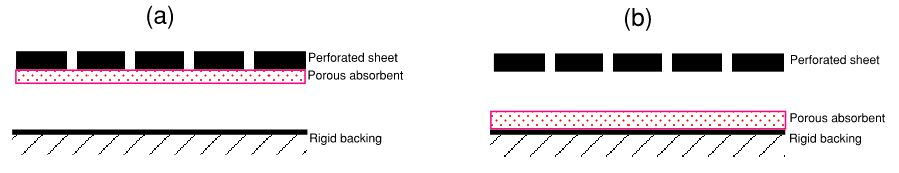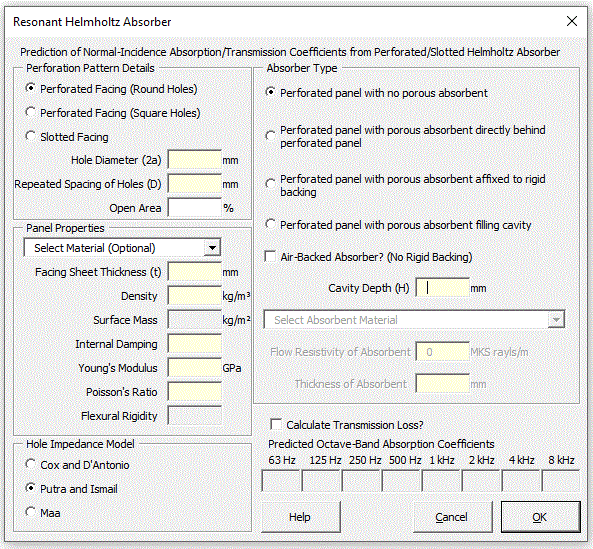### Strutt Help

Absorption or Transmission Coefficients of Resonant Helmholtz Absorber   1/1,1/3

Strutt|Building Acoustics|Resonant (Helmholtz) Absorber calculates the normal-incidence absorption coefficients or transmission loss of a resonant Helmholtz-type absorber implemented as a perforated or slotted sheet over an air gap, and inserts the calculated values into the active row of the worksheet.
Strutt calculates for the following absorber geometries:

• Perforated/slotted sheet on airgap with no porous absorbent
• Perforated/slotted sheet on airgap with porous absorbent directly behind perforated panel (i.e. at top of airgap) as per figure 1(a)
• Perforated/slotted sheet on airgap with porous absorbent directly in front of rigid backing (i.e. at bottom of airgap) as per figure 1(b)
• Perforated/slotted sheet with porous absorbent filling airgapFigure 1

For each absorber configuration, the user may select the absorber to be air-backed (i.e. a free-floating absorber with a semi-infinite airspace behind) in lieu of the default rigid (infinite impedance) backing. This is useful, for example, in calculating the transmission loss (and hence acoustic transparency) of a perforated or slotted facing.Figure 2

Strutt calculates the normal-incidence absorption coefficients alpha from the complex pressure-reflection factor R between the surface of the absorber and the air using the following formula:

R = (Z_S-Z_0)/(Z_S+Z_0)

alpha = 1-|R|^2

where:
Z_0 is the characteristic impedance (rho C) of air
Z_S is the surface impedance of the absorber

With a partitioned-cavity (e.g. a 'honeycomb' type structure behind the perforated panel), the oblique-incidence absorption coefficients will be essentially the same as for normal-incidence. Oblique-incidence coefficients for non-partitioned cavities may be added in a future release.

Strutt calculates the normal-incidence transmission loss using the complex pressure-transmission factor T of the construction and the power transmission coefficient tau using the following formulae:

T = (2Z_S)/(Z_S+Z_0)

tau = |T|^2

"TL" = 10log_"10"(tau)

The surface impedance of the resonant absorber is calculated using:

Z_S = Z_R + Z_(n-1)

where:
Z_R is the effective impedance of the perforated facing

Z_(n-1) is the impedance of the layer of the absorber immediately under the perforated sheet (either air or a porous material).

The facing impedance is calculated using the specific hole impedance z_h and (optionally) the mechanical impedance of the perforated plate z_p as follows:

Where the mechanical properties of the panel are entered, the perforated sheet impedance is the effective impedance (in parallel) of the hole impedance and the mechanical impedance:

Z_R = z_h/(1 + gamma zeta z_h/z_q)
z_q is a modified plate impedance; z_q = z_p + (Re(Z_h)Im(Z_h))/z_h
gamma = 1 - (Im(Z_h))/z_h is a complex non-dimensional term
zeta = 1 - epsilon + (Re(Z_h))/z_h is a complex non-dimensional term

If the hole size is zero (i.e. unperforated sheet), the facing impedance is calculated using only the mechanical impedance of the plate z_p:

Z_R = z_p

If the mechanical properties of the sheet are not entered, the facing impedance is calculated using only the specific hole impedance z_h:

Z_R = z_h

The hole impedance Z_h is calculated using one of three user-selected theoretical models:

• Using the Cox and D'Antonio simplified theory for hole impedance (valid for millimetre-size perforations):

Z_h = rho (t/(2a)+1)sqrt(8 nu omega) + (2 delta a + t) (j omega rho)
• Using the Putra and Ismail theory for hole impedance (valid for both sub-millimetre and millimetre-sized perforations):

Z_h = 32 eta t / ((2a) ^ 2) (sqrt(1 + (X_0 ^ 2) / 32) + (sqrt(2))/8 X_0 (2a) / t) + j rho_0 omega t (1 + 1 / sqrt(9 + (X_0 ^ 2)/2) + delta (2a) / t)

where X_0 = a sqrt((omega rho_0) / eta) is a normalised frequency term
• Using Maa's theory for hole impedance for micro-perforated (sub-millimetre) holes (also cited in Cox and D'Antonio's book):

Z_h=j(omega rho_0 t) (1 - (2 J_1(X_0 sqrt(-j))) / (X_0 sqrt(-j) J_0(X_0 sqrt(-j))))^-1+ sqrt(2 omega rho_0 eta) / 2 + j (1.7 omega rho_0 a)

The first term is the acoustic impedance of a tube that is short compared to wavelength.

The second term is the radiation resistance of an orifice.

The final term is the end correction to the radiation reactance of the tube.

J_0(x) and J_1(x) are the Bessel functions of the first kind.

The specific hole impedance z_h is obtained from the hole impedance Z_h and the perforation ratio epsilon:

z_h = Z_h/epsilon

The in vacuo mechanical impedance z_p of the plate is calculated as follows:

z_p = j omega m(1 - (j mu omega_n)/omega)-(js)/omega

m is the panel surface mass (kg/m2)
s is the panel stiffness. Strutt calculates the stiffness from the mechanical properties of the panel material using a first-order approximation for the flexural rigidity of a plate

s = (E t^3)/(12(1-upsilon^2))
E is the Young's Modulus of the material
upsilon is the Poisson's ratio of the material
t is the plate thickness
The mechanical properties of the plate are by default taken from the materials database used in the Transmission Loss Prediction calculation, but may be modified by the user.
In reality, the edge strutt properties of the absorber will also affect the stiffness (and are not taken into account in the approximate form of the panel stiffness calculation); accordingly use the results of the absorption prediction with caution where the panel absorption component from the plate is predicted to be significant compared to the Helmholtz component.
mu is the mechanical loss factor
omega_n = sqrt(s/m) is the undamped natural frequency of the panel

epsilon is the fractional open area of the perforated sheet

• epsilon = (pi a^2)/d^2; for round perforations

• epsilon = a^2/d^2; for square perforations

• epsilon = a/d; for slotted perforations

a is the hole radius of perforations (circular perforated sheet), hole dimension (square perforated sheet) or slot width (slotted sheet)
d is the repeated spacing of the perforations/slots
t is the thickness of the covering sheet
rho_0 is the density of air (1.21 kg/m3)
eta is the viscosity of air (18.4x10-6 Ns/m2)
nu is the kinematic viscosity of air (15x10-6 m2/s)
delta is an end-correction factor for flow through the slots/perforations:

• delta = {(0.8(1-1.47 sqrt epsilon) \ " for " \ epsilon <= 0.16
\ ),(0.8(1-1.47 epsilon^0.5+0.47 epsilon^1.5) \ " for " epsilon > 0.16 \ ):} for round perforations

• delta = {(0.85(1-1.25 sqrt epsilon) \ " for " \ epsilon <= 0.16
\ ),(0.85(1-1.25 epsilon^0.5+0.47 epsilon^1.5) \ " for " epsilon > 0.16 \ ):} for square perforartions

• delta = -1/pi ln[sin(0.5 pi epsilon)] for slotted perforations

omega is the angular frequency (2 pi f)
j is the square root of -1.

The surface impedance of one layer of the absorber (Z_(Si+1)) is related to the surface impedance of the layer below it (Z_(Si)) using:

Z_(Si+1) = (-j Z_(Si) Z_i cot(k_(x i) d_i) + Z_i^2)/(Z_(Si) - j Z_i cot(k_(x i) d_i))

where:
d_i is the thickness of the layer
Z_i is the characteristic impedance of the layer
k_(x i) is the wavenumber for normal-incidence sound propagation in the layer

The surface impedance of the bottom layer of the absorber is found using the above formula, but imposing either:

• a boundary condition where the lower surface is rigid (and therefore has infinite impedance). In this case the formula reduces to:

Z_1 = -j Z_i cot(k_(x i) d_i)
• a boundary condition where the absorber is backed by a semi-infinite airspace, in which case:

Z_(Si)=Z_0

For air, Z_i = Z_0 and k_(x i) = k_0 = omega//c

For porous absorbent, Z_i and k_(x i) are calculated using the Delaney and Bazley formulation:

Z_i = rho_0 c_0 (1 + 0.0571X^(-0.754) - j 0.087X^(-0.732))

k = k_0 (1 + 0.0978X^(-0.7)-j0.189X^(-0.595))

where X is a frequency-dependent parameter given by:

X = (rho_0 f)/sigma

sigma is the flow resistivity (MKS rayls/m) of the porous absorbent.

A drop-down menu is provided giving flow-resistivity values for several common absorbent materials, or else the user may enter in the flow resistivity manually.

Strutt calculates the octave-band absorption coefficient as the aritmetic average of 50 geometrically-spaced points within each octave-band. A sensitivity study was conducted and 50 sample points in each octave were found to give an acceptable value for the octave-band absorption coefficient, being within 4 significant figures of the value produced by taking 1000 samples in each octave band.

References:

• T.J. Cox and P. D'Antonio (2009) Acoustic Absorbers and Diffusers: Theory, Design and Application 2nd Edition, pp209-227 Spon Press
• A. Putra and A. Y. Ismail (2014), Normal Incidence of Sound Transmission Loss from Perforated Plates with Micro and Macro Size Holes, Advances in Acoustics and Vibration, 2014, Article ID 534569, 12 pages, 2014.
http://www.hindawi.com/journals/aav/2014/534569/cta/
• D.-Y. Maa (1998) Potential of microperforated panel absorber, J Acoust Soc Am, 1998, 104(5):2861-6.